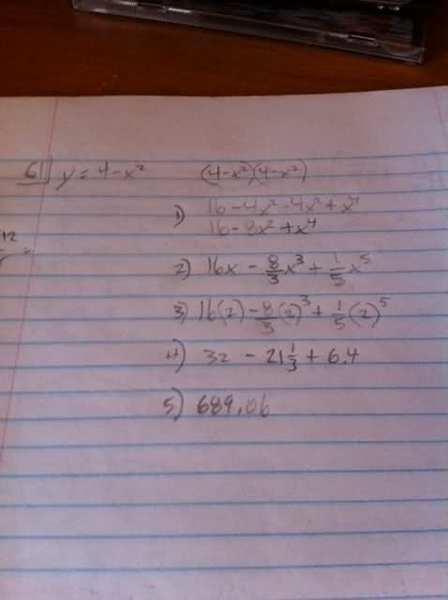# Finding volume of area revolved around x axis

## Homework Statement

The area bounded by the x-axis and curve
y = 4-x^2

is rotated about x-axis, find the volume.

## Homework Equations

[integral] pi y^2 dx

## The Attempt at a Solutionthe answer is supposed to be 512/15 pi

SammyS
Staff Emeritus
Homework Helper
Gold Member

## Homework Statement

The area bounded by the x-axis and curve
y = 4-x^2

is rotated about x-axis, find the volume.

## Homework Equations

[integral] pi y^2 dx

## The Attempt at a Solutionthe answer is supposed to be 512/15 pi

That's 1/2 of the given correct answer.

Where does y = 4-x2 intercept the x-axis ?

[If you know how to find the volume of solid of revolution then you can skip to the equations at the bottom of my post.]

Ok, first go through this website:

http://curvebank.calstatela.edu/volrev/volrev.htm
As you can see, when you revolve a curve about the x-axis, you get a bunch of circles stacked on top of each other. The radius of each circle is equal to the height from the x-axis to the edge of the circle, which is the y-value at that point of the curve. Thus, the formula becomes pi * f(x)^2 with an integral having limits which depend on how much of the curve you revolve.

If you graph the curve, (I cheated and used the grapher app on mac), you can find and locate the region bounded by the curve and the x axis. You will be able to see that the points where the curve touches the x-axis are none other than the roots of the quadratic equation.

To recap:

For f(x), use the equation 4-x^2

For the limits, solve the quadratic equation for the x intercepts, and use those as the limits.

Finally, solve the integral with the limits as the x intercepts, and the function as 4-x^2.

$\int _{ intercept1 }^{ intercept2 }{ \pi (4-{ x }^{ 2 })\quad dx }$

[[STRIKE]I'm too lazy to find the roots.[/STRIKE] Finding the roots will be good practice for you and should be easy if you've passed algebra I]

Last edited:
I could have answered that easily. Zeros are 2 and -2.

HallsofIvy
Homework Helper
You appear to have integrated from 0 to 2. But nothing is said about the y-axis being a boundary.

Also, you leave $\pi$ out until the last step. That's very confusing.

Understood. I did integrate with 2 only. So I should be integrating from -2 to 2. I quickly did that calculation and came up with -48.93333 using the -2. That can't be right.

so you're saying that -2 and 2 shouldn't be used since the y axis is not a boundary?

EDIT: I got it, integrating -2 answer -17.067

Last edited:
SammyS
Staff Emeritus